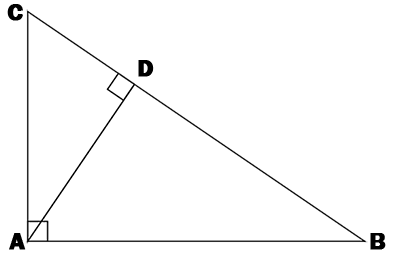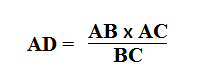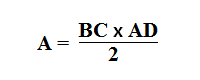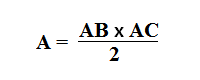### Calculate the area of a right triangle if the hypotenuse of the triangle is 19 cm long and the height 12 cm long.Fill in herecm2

### Remember !

A right triangle is the right-angled triangle. The side opposite the right angle is called the hypotenuse and the other two sides are called the cathetus.

Abbreviations: A - area, AB - cathetus, AC - cathetus, BC - hypotenuse and AD - height.Height formula in a right triangle.The formula of the area of the right triangle.Abbreviations: A - area, AB - cathetus, AC - cathetus, BC - hypotenuse and AD - height.

### Exemple !

1. The area of a right triangle is 60 cm² and the height AD is 8 cm long. Calculate the length of the hypotenuse.

60 = (BC × AD) / 2

60 = (BC × 8) / 2

120 = BC × 8

BC = 15

2. Calculate the area of a right triangle if the beads are 10 cm long and 6 cm long.

A = (AB × AC) / 2

A = (10 × 6) / 2

A = 60 : 2

The area is 30 cm²

3. Calculate the area of a right triangle if the hypotenuse of the triangle is 12 cm long and the height 7 cm long.

A = (BC × AD) / 2

A = 84 : 2

The area is 42 cm²

Site-ul nostru poate rămâne gratuit numai prin afișarea de reclame.

Vă rugăm să ne susțineți prin dezactivarea blocării de anunțuri.